+关注继续查看

# 神经网络案例

## 摘要

• 在Compute Vision（计算机视觉）中，我们输入的是一张一张的图片，但是在计算机看来，每一张图片都是由一个一个像素点组成的，那么，什么是我们的输入样本X，什么又是我们的标签y？在图像识别中，一张图片所有像素点就是一个样本，也就是矩阵X中的一行，y就是对这个图片判断的结果。可想而知，就算是一张50x50的图片，它的特征也有2500之多，如果他是RGB图片，那就是7500个特征，那么特征的值是什么？就是图片的亮度（intensity），它的是在0-255之间。在图像识别中，我们会将像素矩阵unrolled成为一个向量，将这个向量作为一个训练集。如果要可视化图片，需要将其在转换为矩阵。
• activation function（激活函数），其实它就是在机器学习领域中的sigmoid（logistic）函数，这是在神经网络中换了一个名字罢了。
• 在深度学习中，我们会遇到比较复杂的$J(\theta)$目标函数(cost function), 一般情况下，使用梯度下降的方法来计算$J(\theta)$目标函数的最小值，有下面这个梯度下降公式$\theta := \theta - {{\partial}\over{\partial{\theta}}}J(\theta)$其实${{\partial}\over{\partial{\theta}}}J(\theta)$是梯度，梯度下降公式的关键点就是计算出梯度，在线性回归和逻辑回归这些简单的模型中的目标函数的梯度是好求的，可以直接带入偏导的公式，但是在深度学习中比较复杂，求梯度需要回归到最原始的求法，使用定义法进行求导，不过这样的计算量非常的大，于是后来就诞生了反向传播算法(backpropagation algorithm)，这个算法大量地使用了链式求导法则。大致上是下面的公式(如果实现了反向传播算法，要通过梯度检测，之后再投入到训练中，因为反向传播算法比较的复杂，实现起来可以会有bug)
• 假设我们现在有4个layer，输出层是一个节点(这是一个单分类的问题)
• $\delta^{(4)}=(a^{(4)}-y)$
• $\delta^{(3)}=({\theta^{(3)}})^{T}{\delta}^{(4)} \times g(z^{(3)})$, 其中$\delta^{(3)}$, $z^{(3)}$等都是向量或者矩阵，建议在数学公式推导的时候使用实数，在推导结束时候，放到matlab等应用的时候将其转为向量或者矩阵表示。
• $\delta^{(2)} = ({\theta^{(2)}})^{T}{\delta}^{(3)} \times g(z^{(2)})$
• 第一层是不需要计算误差的，因为它是我们原始的输入层。
• 上述式子中的$g(z^{(3)})$等于sigmoid($z^{(3)}$)(sigmoid($z^{(3)} - 1$))
• 在上面我们已经知道了，在使用了反向传播算法计算出误差值(error)，为了防止使用反向传播高级算法出现bug，需要使用正规的求导的方法来检测反向传播算法是否出现了bug，只要gradApprox $\approx$ DVec则表示没有bug，对于如何实现梯度检测，只需要构建一个小型的神经网络，接着生成一些数据X和标签y分别输入到反向传播函数和一般计算梯度的函数中，最后将结果进行比较即可
• 在神经网络中会有非常多的参数，为这些参数赋予初始值是非常重要的，于是就产生了随机初始化的方法(random initialize), 一个参数表示为$\theta_{ji}^{(l)}$，随机初始化的目的就是将$\epsilon$的初始值在$-\epsilon$$\delta$之间
• 在进行反向传播的时候，计算出来的error item $\delta$ 的个数与参数的个数一样的，因为error item与每一个与之对应的参数密切相关，我们要通过error item计算出参数的梯度theta_grad，当所有的训练样本都输入进去并参与到了error item的运算中去的时候，得到的theta_grad与参数是同维度的，由此可以推断出，error item 也是与参数同维度的，这里将error item $\approx {{\partial}\over{\partial{\theta}}}J(\theta)$\$，这样就计算出了梯度，接下来就可以更新参数了
• 我们知道在神经网络中我们的参数$\theta$成为了多个矩阵，返回的梯度也成为多个矩阵，这与我们之前学习的线性回归和逻辑回归不同，他们的是一个列向量，上面提到的是在一次迭代中，因此为了统一和方便编程，将几个矩阵全部unrolled成为一个列向量

## 规定

• L: 表示layer的个数
• $a_{i}^{(j)}$: 表示第j层layer的第i个单元(unit)
• $s_{j}$: 表示第j层layer的单元的个数
• $\Theta^{(j)}$: 表示第j层layer的权重矩阵

## 代码

• costFunction

% 将y标签的值转为[0 0 0 0 1 0 0 0 0 0 ...]的形式
% 使用for循环迭代每一个样本
% 注意，显示的情况就是参数都已经有了，在一个for循环中，输入的是一个样本
tmp = (1:num_labels)';
for i = 1:m
% 将其中的一个样本的标签转化为[0 0 0 0 0 1 ... 0 0]形式
% 神经网络的前向传播
y_new = y(i) == tmp;
x = [1 X(i, :)];
z_2 = Theta1 * x';
a_2 = sigmoid(z_2); % 25x1 demensions
z_3 = Theta2 * [1, a_2']';
a_3 = sigmoid(z_3);
% 计算损失函数
J = J + sum(-y_new .* log(a_3) - (1 - y_new) .* log(1 - a_3));

% 神经网络的后向传播
delta_3 = a_3 - y_new;
delta_2 = Theta2' * delta_3 .* [1; sigmoidGradient(z_2)];
delta_2 = delta_2(2:end);

end
J = 1 / m * J;
J = J + lambda / (2 * m) * (sum(sum(Theta1 .^ 2)) + sum(sum(Theta2 .^ 2)));
% =========================================================================

Theta2_grad(:, 1) = Theta2_grad(:, 1) - lambda / m * Theta2(:, 2);

end
• randomInitWeights
% You need to return the following variables correctly
W = zeros(L_out, 1 + L_in);

% ====================== YOUR CODE HERE ======================
% Instructions: Initialize W randomly so that we break the symmetry while
%               training the neural network.
%
% Note: The first column of W corresponds to the parameters for the bias unit
%
epsilon_init = 0.12;
W = rand(L_out, L_in + 1) * 2 * epsilon_init - epsilon_init;

% =========================================================================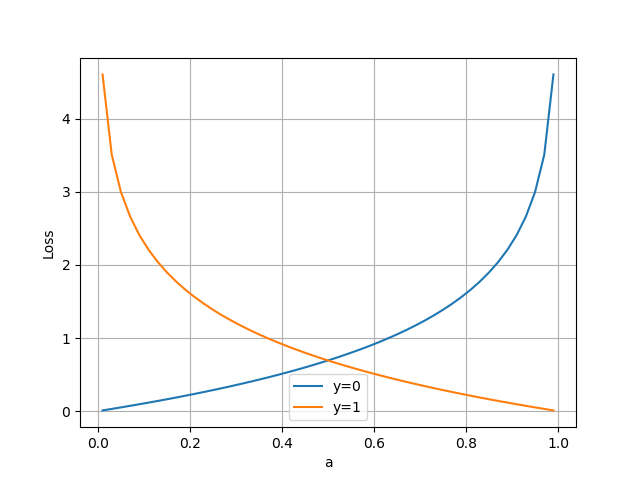119 0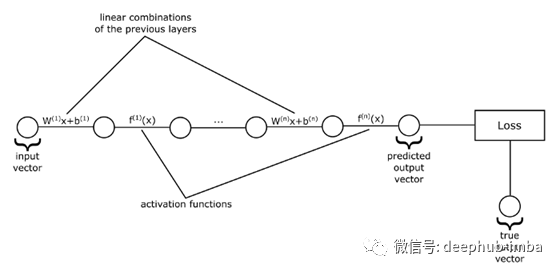40 0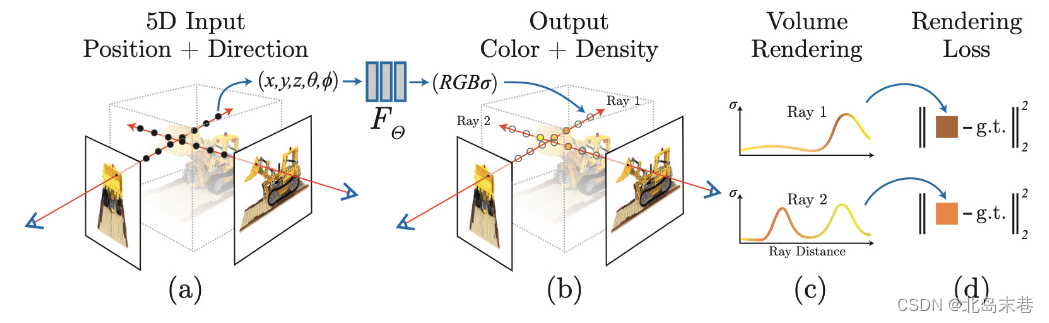NeRF神经网络介绍

610 0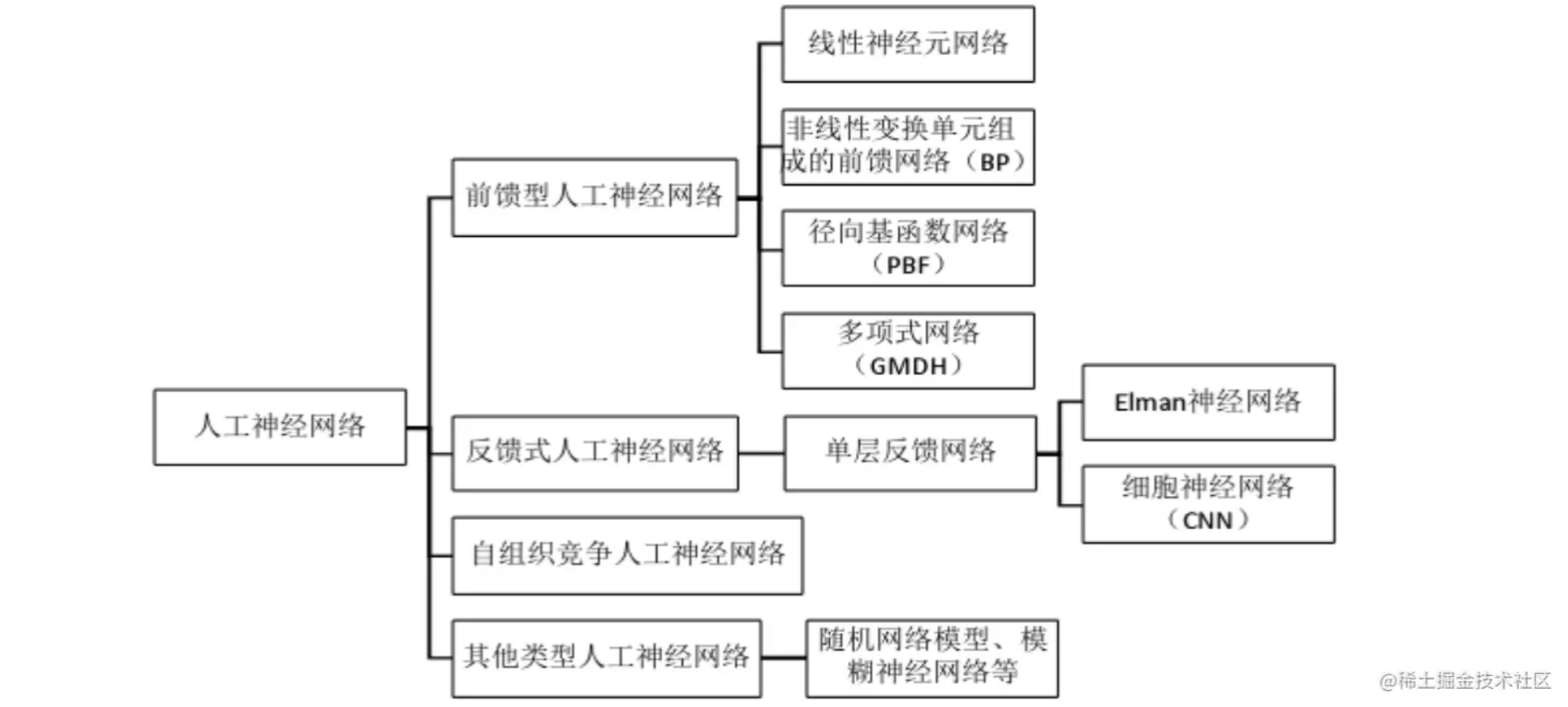273 0227 0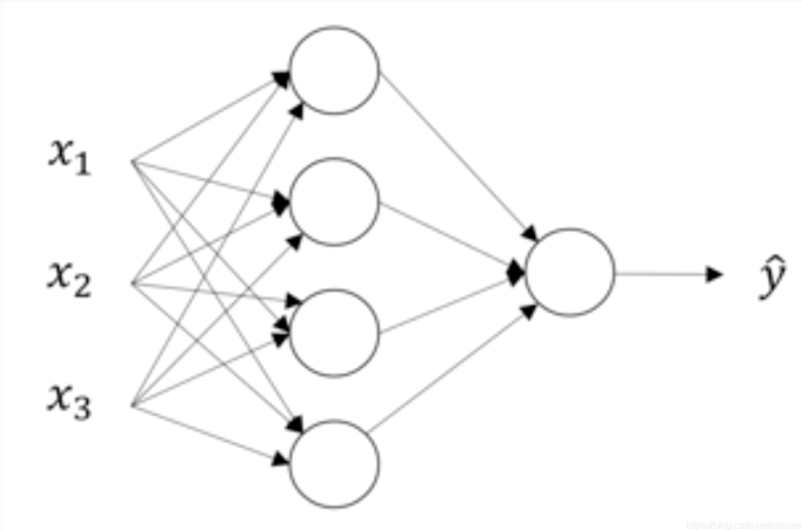58 0
megachen
Just for fun

【算法实战】6. 支持向量机算法5610